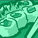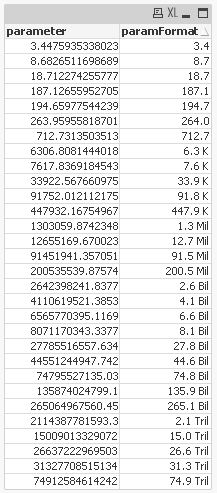# QlikView App Dev

Discussion Board for collaboration related to QlikView App Development.

Announcements
Support Case Portal has moved to Qlik Community! Read the FAQs to start exploring Support resources.
cancel
Showing results for
Did you mean:Specialist

## dynamically changing scale in text box

Hi qlikgurus,

I need to a logic for dynamic scaling , such as

in text box we showing                 total revenue ,

incremental profit

gross units sold

as values are very big we try to show them in billions but upon selections and to see granular data i need to come up with dymanic scale

have any here came up this kind of problem is there a way of writing expression such that based on selctions the symbols chnges from Billion to million to thousand

thanks have a good day

1 Solution

Accepted SolutionsMVP

Maybe you could do it like this:

=num( sum( revenue) /pow(1000,floor(log10(fabs(sum(revenue)))/3)),'##0.000')

& pick(floor(log10(fabs(sum(revenue)))/3)+1,'',' thousand',' million',' billion',' trillion')

i.e. dividing your revenue value by some appropriate number and adding thousand, million, billion or trillion (or any other appropriate postfix).

Hope this helps,

Stefan

7 RepliesMVP

Maybe you could do it like this:

=num( sum( revenue) /pow(1000,floor(log10(fabs(sum(revenue)))/3)),'##0.000')

& pick(floor(log10(fabs(sum(revenue)))/3)+1,'',' thousand',' million',' billion',' trillion')

i.e. dividing your revenue value by some appropriate number and adding thousand, million, billion or trillion (or any other appropriate postfix).

Hope this helps,

StefanSpecialist
Author

thanks stefans........appreciate it

will let you know once it's worked

have a great daySpecialist
Author

wow !!!  super stefan it's working great

and is thera way we can use them in chart

say suppose we have straight with values ranging for billions how change scale dynamicallu for those values as we did hereMVP

Not sure if I understand your setting, the expression should just work fine in a straight table chart expression.

I believe one limitation is that the expression will return a string, so you can only put it in where a string as result makes sense (for example, I don't think you can use it as bar chart expression, but you can use a bar chart with first expression sum( revenue) and display option bars, second expression like above with display options 'values on data points' and bars deselected. Also you might want to enable a logarithmic 'log scale' in axis tab).

Regards,

StefanSpecialist
Author

thankyou very much stefanMVP

maybe one possibility to avoid the number-as-string limitation:

```SET vSize = Div(Log10(\$1),3);
SET vFormatNumber = Dual(Num(\$1/Pow(10,3*\$(vSize(\$1))),'#,##0.0')&' '&Pick(\$(vSize(\$1)),'K','Mil','Bil','Tril'),\$1);

\$(vFormatNumber(parameter)) as paramFormat;
AutoGenerate 30;
```regards

MarcoNot applicable

If you want to achieve this in the front end and keep the numeric format, place the symbol part (K,M,B) inside the number format string definition.

NUM(

SUM( [MeasureField] )

/

POW(1000 ,FLOOR(LOG10(FABS(SUM([MeasureField])))/3))

,'##0.0' & PICK(FLOOR(LOG10(FABS(SUM([MeasureField])))/3)+1,'',' K',' M',' B'))

RegardsCommunity Browser